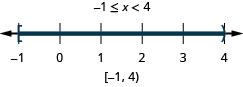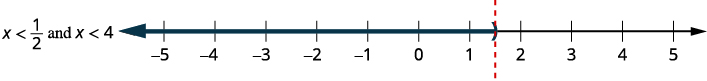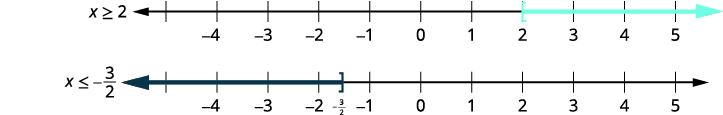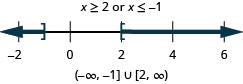# 2.7：求解复合不等式

•• OpenStax
• OpenStax
$$\newcommand{\vecs}{\overset { \rightharpoonup} {\mathbf{#1}} }$$ $$\newcommand{\vecd}{\overset{-\!-\!\rightharpoonup}{\vphantom{a}\smash {#1}}}$$$$\newcommand{\id}{\mathrm{id}}$$ $$\newcommand{\Span}{\mathrm{span}}$$ $$\newcommand{\kernel}{\mathrm{null}\,}$$ $$\newcommand{\range}{\mathrm{range}\,}$$ $$\newcommand{\RealPart}{\mathrm{Re}}$$ $$\newcommand{\ImaginaryPart}{\mathrm{Im}}$$ $$\newcommand{\Argument}{\mathrm{Arg}}$$ $$\newcommand{\norm}{\| #1 \|}$$ $$\newcommand{\inner}{\langle #1, #2 \rangle}$$ $$\newcommand{\Span}{\mathrm{span}}$$ $$\newcommand{\id}{\mathrm{id}}$$ $$\newcommand{\Span}{\mathrm{span}}$$ $$\newcommand{\kernel}{\mathrm{null}\,}$$ $$\newcommand{\range}{\mathrm{range}\,}$$ $$\newcommand{\RealPart}{\mathrm{Re}}$$ $$\newcommand{\ImaginaryPart}{\mathrm{Im}}$$ $$\newcommand{\Argument}{\mathrm{Arg}}$$ $$\newcommand{\norm}{\| #1 \|}$$ $$\newcommand{\inner}{\langle #1, #2 \rangle}$$ $$\newcommand{\Span}{\mathrm{span}}$$$$\newcommand{\AA}{\unicode[.8,0]{x212B}}$$

##### 学习目标

• 用 “and” 求解复合不等式
• 用 “或” 求解复合不等式
• 求解具有复合不等式的应用程序

1. 简化：$$\frac{2}{5}(x+10)$$
如果你错过了这个问题，请查看 [链接]
2. 简化：$$−(x−4)$$
如果你错过了这个问题，请查看 [链接]

## 用 “and” 求解复合不等式

$\begin{array} {lll} {x+3>−4} &{\text{and}} &{4x−5\leq 3} \\ {2(y+1)<0} &{\text{or}} &{y−5\geq −2} \\ \end{array} \nonumber$

##### 复合不等式图$$\PageIndex{1}$$

$$−3$$该数字在第一张图上没有阴影，因此由于两张图上都没有阴影，因此它不包含在解图中。

##### 示例$$\PageIndex{1}$$

 $$6x−3<9$$ 和 $$2x+9\geq 3$$ 第 1 步。 解决每个 不等式。 $$6x−3<9$$ $$2x+9\geq 3$$ $$6x<12$$ $$2x\geq −6$$ $$x<2$$ 和 $$x\geq −3$$ 第 2 步。 绘制每个解决方案的图表。 然后绘制使两个不等式都成真的数字。 最后一张图将显示使两个不等式都成真的所有数字，即前两张图上的阴影数字。第 3 步。 用间隔表示法写出解。 $$[−3,2)$$ 使两个不等式都成真的所有数字都是复合不等式的解决方案。
##### 示例$$\PageIndex{2}$$##### 示例$$\PageIndex{3}$$##### 用 “AND” 求解复合不等式。
1. 解决每个不等式。
2. 绘制每个解决方案的图表。 然后绘制使两个不等式都成真的数字的图形。
这张图显示了复合不等式的解。
3. 用间隔表示法写出解。
##### 示例$$\PageIndex{4}$$

 $$3(2x+5)\leq 18$$ 和 $$2(x−7)<−6$$ 解决每个 不等式。 $$6x+15\leq 18$$ $$2x−14<−6$$ $$6x\leq 3$$ $$2x<8$$ $$x\leq \frac{1}{2}$$ 和 $$x<4$$ 绘制每个 解决方案的图表。绘制使两个 不等式都成真的数字 。用间隔表示法写出解。 $$(−\infty, \frac{1}{2}]$$
##### 示例$$\PageIndex{5}$$##### 示例$$\PageIndex{6}$$##### 示例$$\PageIndex{7}$$

 $$\frac{1}{3}x−4\geq −2$$ 和 $$−2(x−3)\geq 4$$ 解决每个不等式。 $$\frac{1}{3}x−4\geq −2$$ $$−2x+6\geq 4$$ $$\frac{1}{3}x\geq 2$$ $$−2x\geq −2$$ $$x\geq 6$$ 和 $$x\leq 1$$ 绘制每个解决方案的图表。绘制 使两个不等式都成 真的数字。没有数字可以证明这两个不等式都是正确的。 这是矛盾的，所以没有解决方案。没有数字可以证明这两个不等式都是正确的。 这是矛盾的，所以没有解决方案。没有数字可以证明这两个不等式都是正确的。 这是矛盾的，所以没有解决办法。
##### 示例$$\PageIndex{8}$$##### 示例$$\PageIndex{9}$$##### 双重不平等

$\text{Other forms:} \quad \begin{array} {lllll} {a<x<b} &{\text{is equivalent to }} &{a<x} &{\text{and}} &{x<b} \\ {a\leq x\leq b} &{\text{is equivalent to }} &{a\leq x} &{\text{and}} &{x\leq b} \\ {a>x>b} &{\text{is equivalent to }} &{a>x} &{\text{and}} &{x>b} \\ {a\geq x\geq b} &{\text{is equivalent to }} &{a\geq x} &{\text{and}} &{x\geq b} \\ \end{array} \nonumber$

##### 示例$$\PageIndex{10}$$

 $$-4 \leq 3x - 7 < 8$$ 在所有三个部分中加上 7。 $$-4 \,{\color{red}{+\, 7}} \leq 3x - 7 \,{\color{red}{+ \,7}} < 8 \,{\color{red}{+ \,7}}$$ 简化。 $$3 \le 3x < 15$$ 将每个部分除以三。 $$\dfrac{3}{\color{red}{3}} \leq \dfrac{3x}{\color{red}{3}} < \dfrac{15}{\color{red}{3}}$$ 简化。 $$1 \leq x < 5$$ 绘制解决方案的图表。用间隔表示法写出解。 $$[1, 5)$$

##### 示例$$\PageIndex{11}$$##### 示例$$\PageIndex{12}$$## 用 “或” 求解复合不等式

##### 用 “OR” 求解复合不等式。
1. 解决每个不等式。
2. 绘制每个解决方案的图表。 然后绘制使任一不等式都成真的数字的图表。
3. 用间隔表示法写出解。
##### 示例$$\PageIndex{13}$$

 $$5−3x\leq −1$$ 要么 $$8+2x\leq 5$$ 解决每个不等式。 $$5−3x\leq −1$$ $$8+2x\leq 5$$ $$−3x\leq −6$$ $$2x\leq −3$$ $$x\geq 2$$ 要么 $$x\leq −\frac{3}{2}$$ 绘制每个解决方案的图表。使任一不等式都 成真的图形数字。$$(−\infty,−32]\cup[2,\infty)$$
##### 示例$$\PageIndex{14}$$##### 示例$$\PageIndex{15}$$##### 示例$$\PageIndex{16}$$

 $$\frac{2}{3}x−4\leq 3$$ 要么 $$\frac{1}{4}(x+8)\geq −1$$ 解决每个 不等式。 $$3(\frac{2}{3}x−4)\leq 3(3)$$ $$4⋅\frac{1}{4}(x+8)\geq 4⋅(−1)$$ $$2x−12\leq 9$$ $$x+8\geq −4$$ $$2x\leq 21$$ $$x\geq −12$$ $$x\leq \frac{21}{2}$$ $$x\leq \frac{21}{2}$$ 要么 $$x\geq −12$$ 绘制每个 解决方案的图表。使任一 不等式都成真的图形数字 。该解决方案涵盖所有实数。 $$(−\infty ,\infty )$$
##### 示例$$\PageIndex{17}$$##### 示例$$\PageIndex{18}$$## 使用复合不等式求解应用程序

##### 示例$$\PageIndex{19}$$

 确定我们在寻找什么。 他可以使用的HCF数量并保持在 “正常使用” 计费范围内。 说出我们要找的东西。 假设 x=x= 他能使用的 hcf 的数量。 转化为不平等。 账单是24.72美元，再加上他使用的hcf数量的1.54倍或$$24.72+1.54x$$。 $$\color{Cerulean}{\underbrace{\color{black}{\text{His bill will be between or equal to }57.06\text{ and }171.02.}}}$$ $$57.06 \leq 24.74 + 1.54x \leq 171.02$$ 解决不平等问题。 $$57.06 \leq 24.74 + 1.54x \leq 171.02$$ $$57.06 \,{\color{red}{- \,24.72}}\leq 24.74 \,{\color{red}{- \,24.72}} + 1.54x \leq 171.02 \,{\color{red}{- \,24.72}}$$ $$32.34 \leq 1.54x \leq 146.3$$ $$\dfrac{32.34}{\color{red}{1.54}} \leq \dfrac{1.54x}{\color{red}{1.54}} \leq \dfrac{146.3}{\color{red}{1.54}}$$ $$21 \leq x \leq 95$$ 回答问题。 业主可以使用$$21–95$$ hcf，但仍在 “正常使用” 计费范围内。

• 复合不等式

## 关键概念

• 如何用 “and” 求解复合不等式
1. 解决每个不等式。
2. 绘制每个解决方案的图表。 然后绘制使两个不等式都成真的数字的图形。 这张图显示了复合不等式的解。
3. 用间隔表示法写出解。
• 双重不等式
• 双重不等式是一种复合不等式，例如$$a<x<b$$。 它等同于$$a<x$$$$x<b.$$

和其他形式：\ [\ begin {align*} a<x<b &\ text {等同于} & a<x\;\ text {和}\; x<b\\
a≤b &\ text {等同于} & a≤x\;\ text {和}\; x≤b\\
a>x>b &\ text {等同于} & a>x\;\ text {和}\; x>b\\
a≥x≥b &\ text {等同于} & a≥x\;\ text {和}\; x≥b\ end {align*}\]
• 如何用 “或” 求解复合不等式
1. 解决每个不等式。
2. 绘制每个解决方案的图表。 然后绘制使任一不等式都成真的数字的图表。
3. 用间隔表示法写出解。